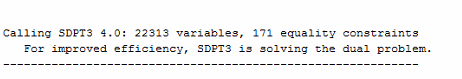# What is the meaning of the number of variables given in Matlab Command window

When I solved a SDP problem, the number of variables shown in Matlab Command window is 22313 (see the figure), but actually I just declared 509 scalar variables belonging to some matrix variables. I was wondering why there is such a huge difference. It seems that inceasing the number of constraints (LMIs in my problem) will increase the number of variables shown in Matlab command window. Hope someone can give me some hints. Thanks a lot.CVX performs a variety of transformations on the problems you enter into it, in order to convert it into a form that the underlying solvers can handle. These transformations sometimes include the creation of new variables and constraints.

Thank you Michael. Got it!

Hey Michael, sorry for reopening this conversation. I tried to find information about these transformations but I couldn’t find it. Could you point me in the right direction?
Thanks!

Try reading this material https://web.stanford.edu/~boyd/papers/disc_cvx_prog.html .

1 Like